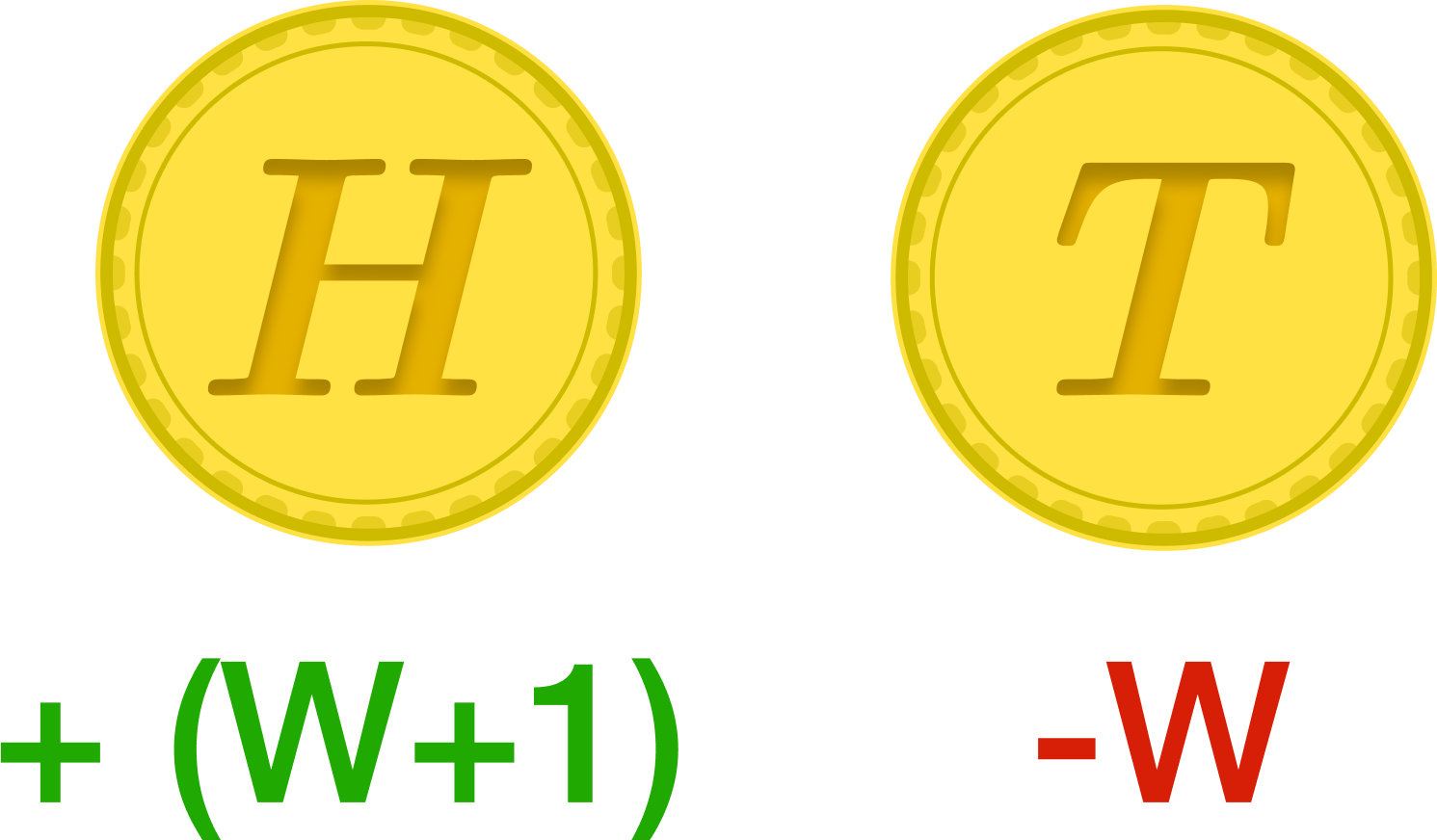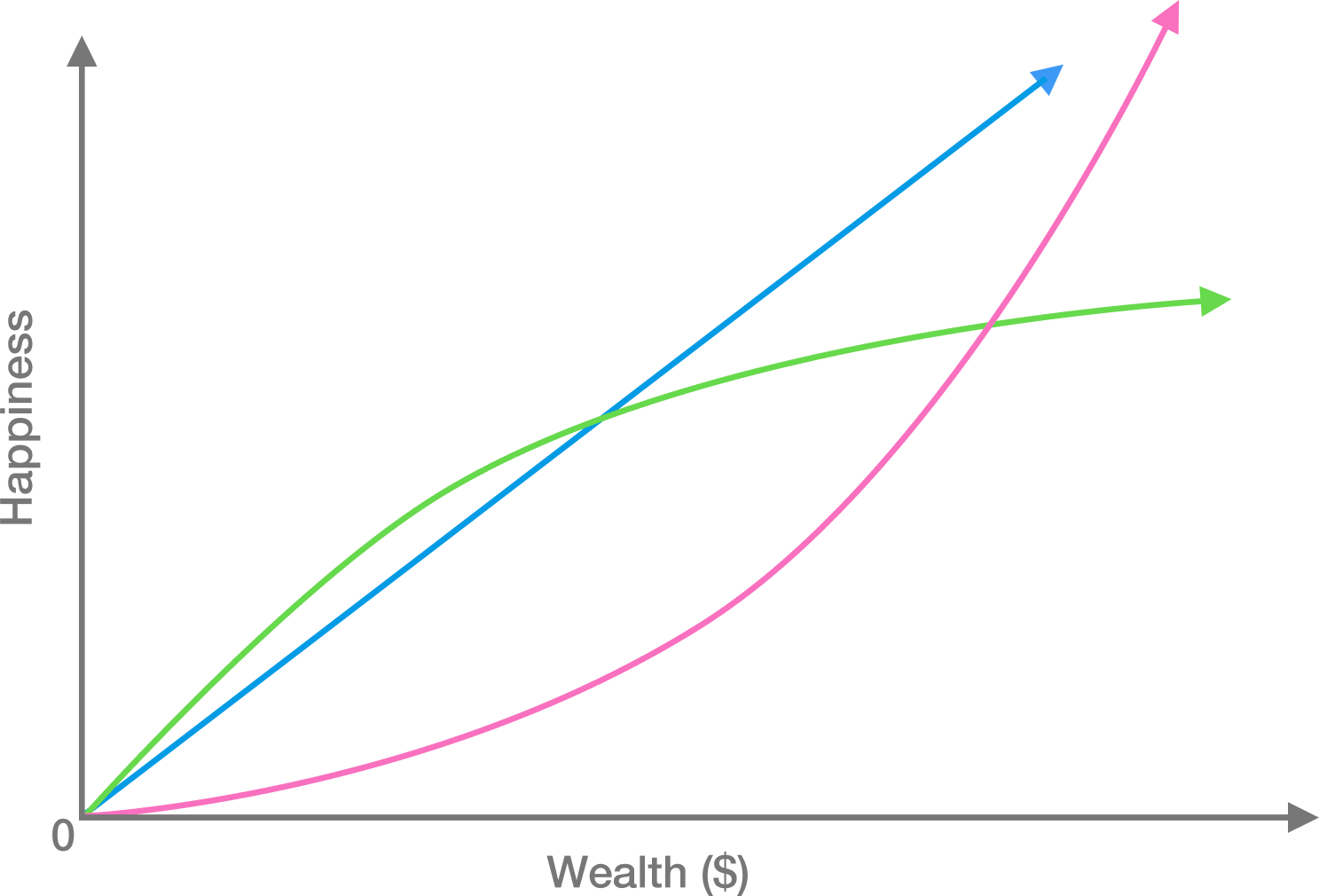### Math for Quantitative Finance

Most people think of value as being measured in terms of money (e.g., dollars). In other words, people and investors make decisions to maximize the expected value of their money. While this is generally true, it is potentially misleading because it does not account for risk.

# Value and RiskIn a one-time bet, a fair coin is flipped. If it is heads, the player doubles their life savings and gets an additional $1. If the coin is tails, they lose all of their assets (their entire life savings, home, etc.). Would the average adult human take this bet? # Value and Risk The last question illustrated that “value” is often more complicated than an expected value calculation. Which of the following curves is a good depiction of an average individual’s “happiness” as a function of their wealth?# Value and Risk A trading firm has the utility function $U(w) = \sqrt{w}$ where $w$ is the wealth of the firm, in dollars. Currently, the firm is worth$100,000,000, so their happiness is $U(100,000,000) = \sqrt{100,000,000} = 10,000,$ and they always want to maximize their expected happiness.

They are offered a risky bet which will succeed with probability $p,$ doubling the wealth of the firm. However, if it fails, the firm will go bankrupt.

Find the smallest probability under which they would take this bet, and then round that probability to the nearest 10%.

Hint: Find their expected utility based on the possibilities after this bet, and determine the conditions under which it exceeds their current utility of $10,000.$

# Value and Risk

The previous question illustrated the idea that “money now is worth more than money later”. A fundamental reason behind this is that money can be invested - in financial assets or elsewhere - so that it grows over time. This is why lenders are paid interest.

If money is lent with an annual interest rate of 1% compounded continuously, about how long would it take for the money to double?

×